# Block cross-validation for species distribution modelling

## Introduction

Simple random selection of training and testing folds in the structured environment leads to an underestimation of error in the evaluation of spatial predictions and may result in inappropriate model selection (Telford and Birks, 2009; Roberts et al., 2017). The use of spatial and environmental blocks to separate training and testing sets has been suggested as a good strategy for realistic error estimation in datasets with dependence structures, and more generally as a robust method for estimating the predictive performance of models used to predict mapped distributions (Roberts et al., 2017). Package blockCV provides functions to separate train and test sets using buffers, spatial and environmental blocks. It provides several options for how those blocks are constructed. It also has a function that applies geostatistical techniques to investigate the existing level of spatial autocorrelation in the covariates to inform the choice of a suitable distance band by which to separate the data sets. In addition, some visualization tools are provided to help the user choose the block size and explore generated folds. The package has been written with Species Distribution Modelling (SDM) in mind, and the functions allow for a number of common scenarios (including presence-absence and presence-background species data, rare and common species, raster data for predictor variables). Although it can be applied to any spatial modelling e.g. multi-class responses for remote sensing image classification.

You can find more information about blocking strategies of blockCV package and in general block cross-validation technique in the package associated paper here.

This document presents the main functions of the package and illustrates its usage with three examples: modelling using randomForest, maxnet (new implementation of Maxent software in R) and biomod2 packages.

## Installation

The blockCV is available in GitHub and it will be available in CRAN soon. It is recommended to install the dependencies of the package. To install the package from GitHub use:

remotes::install_github("rvalavi/blockCV", dependencies = TRUE)
# loading the package
library(blockCV)

## Package data

The package contains the raw format of the following data:

• Raster covariates of Australian Wet Tropic region (.tif)
• Simulated species data (.csv)

These data are used to illustrate how the package is used. The raster data include several bioclimatic and topographic variables from Australian Wet Tropic region aggregated to 800 m resolution. The species data contains records of a species, simulated based on the above environmental variables for the region. There are two .csv files with presence-absence and presence-background data.

To load the package raster data use:

# loading raster library
library(raster)
library(sf)

# import raster data
awt <- raster::brick(system.file("extdata", "awt.grd", package = "blockCV"))

The presence absence species data include 116 presence points and 138 absence points. The appropriate format of species data for the blockCV package is simple features (sf) or SpatialPointsDataFrame. We convert the data.frame to sf as follows:

# import presence-absence species data
PA <- read.csv(system.file("extdata", "PA.csv", package = "blockCV"))
# make a SpatialPointsDataFrame object from data.frame
pa_data <- st_as_sf(PA, coords = c("x", "y"), crs = crs(awt))
# see the first few rows
pa_data
## Simple feature collection with 254 features and 1 field
## geometry type:  POINT
## dimension:      XY
## bbox:           xmin: 262415.8 ymin: 7827168 xmax: 495215.8 ymax: 8300768
## epsg (SRID):    NA
## proj4string:    +proj=utm +zone=55 +south +ellps=GRS80 +units=m +no_defs
## First 10 features:
##    Species                 geometry
## 1        1 POINT (363215.8 8041568)
## 2        1 POINT (352815.8 8062368)
## 3        1 POINT (356815.8 8104768)
## 4        1 POINT (314415.8 8175168)
## 5        1 POINT (356815.8 8028768)
## 6        1 POINT (352015.8 8029568)
## 7        1 POINT (370415.8 8067968)
## 8        1 POINT (435215.8 7878368)
## 9        1 POINT (359215.8 8084768)
## 10       1 POINT (431215.8 7880768)
# plot species data on the map
plot(awt[]) # plot raster data
plot(pa_data[which(pa_data$Species==1), ], pch = 16, col="red", add=TRUE) # add presence points plot(pa_data[which(pa_data$Species==0), ], pch = 16, col="blue", add=TRUE) # add absence points
legend(x=500000, y=8250000, legend=c("Presence","Absence"), col=c(2, 4), pch=c(16,16), bty="n")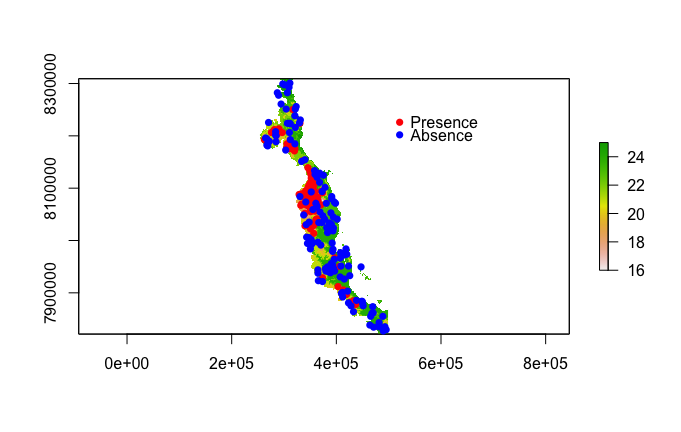The presence background data include 116 presence points and 10,000 random background points (0s here).

# import presence-background species data
PB <- read.csv(system.file("extdata", "PB.csv", package = "blockCV"))
# make a SpatialPointsDataFrame object from data.frame
pb_data <- st_as_sf(PB, coords = c("x", "y"), crs = crs(awt))
# number of presence and background records
table(pb_data$Species) ## ## 0 1 ## 10000 116 ## Blocking strategies ### Spatial block The function spatialBlock creates spatially separated folds based on a pre-specified distance (cell size of the blocks). It then assigns blocks to the training and testing folds with random, checkerboard pattern or in a systematic manner. Another blocking strategy provided by this function is to divide the study area into vertical or horizontal bins of a given number of rows/colmuns, as used by Bahn & McGill (2013) and Wenger & Olden (2012) respectively. To keep the consistency with other functions, the distance (theRange) should be in metres, regardless of the unit of the reference system of the input data. When the input map has geographic coordinate system (decimal degrees), the block size is calculated based on dividing theRange by 111325 (the standard distance of a degree in metres, on the Equator) to change metre to degree. This value can be changed by the user via the degMetre argument. The xOffset and yOffset can be used to shift the spatial position of the blocks in horizontal and vertical axes, respectively. This only works when the block have been built based on theRange. The blocks argument allows users to define an external spatial polygon as blocking layer. The polygon layer must cover all the species points. In addition, blocks can be masked by species spatial data. This option keeps the blocks that cover species data and remove the rest. # spatial blocking by specified range with random assignment sb <- spatialBlock(speciesData = pa_data, species = "Species", rasterLayer = awt, theRange = 70000, # size of the blocks k = 5, selection = "random", iteration = 100, # find evenly dispersed folds biomod2Format = TRUE, xOffset = 0, # shift the blocks horizontally yOffset = 0) ## The best folds was in iteration 48: ## train_0 train_1 test_0 test_1 ## 1 103 99 35 17 ## 2 101 102 37 14 ## 3 108 102 30 14 ## 4 123 105 15 11 ## 5 117 56 21 60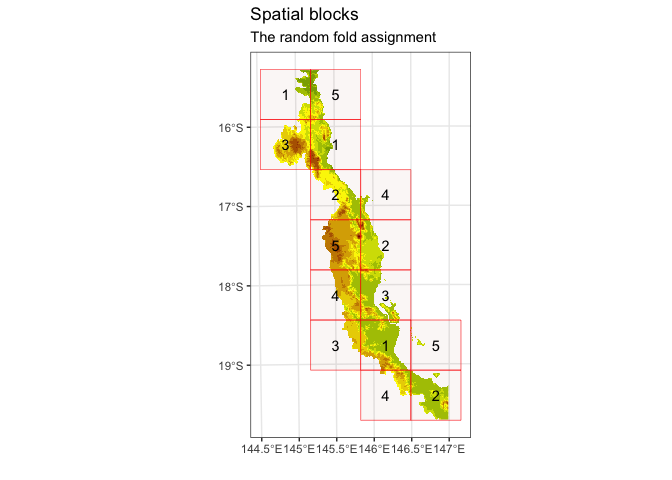# spatial blocking by rows and columns with checkerboard assignment sb2 <- spatialBlock(speciesData = pb_data, # presence-background data species = "Species", rasterLayer = awt, rows = 5, cols = 6, k = 5, selection = "systematic", biomod2Format = TRUE) ## train_0 train_1 test_0 test_1 ## 1 7966 98 2034 18 ## 2 6983 104 3017 12 ## 3 8810 110 1190 6 ## 4 7981 48 2019 68 ## 5 8260 104 1740 12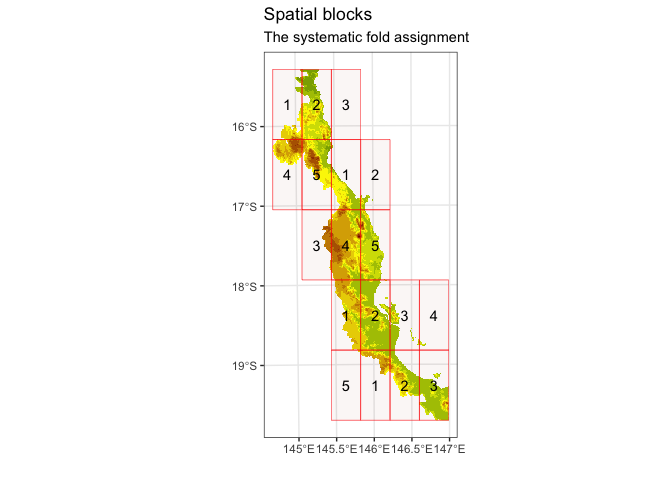The assignment of folds to each block can also be done in a systematic manner using selection = "systematic" argument. # spatial blocking by rows with systematic assignment sb3 <- spatialBlock(speciesData = pa_data, species = "Species", rasterLayer = awt, rows = 6, selection = "checkerboard", biomod2Format = TRUE) ## train_0 train_1 test_0 test_1 ## 1 59 64 79 52 ## 2 79 52 59 64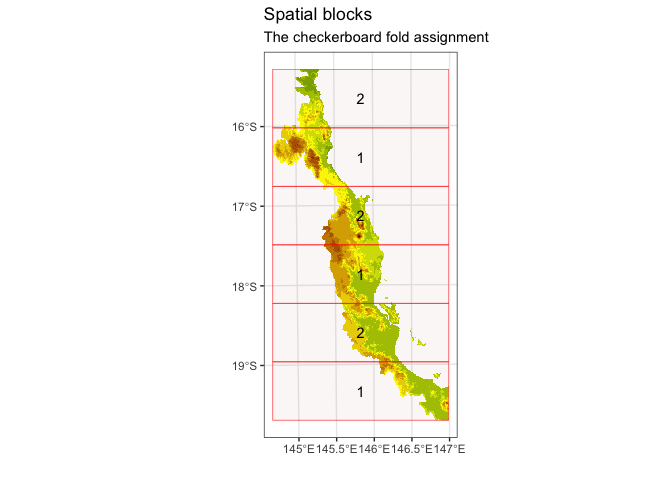For visualising the species data on top of the spatial blocks, one can use geom_sf function of the ggplot2 package. However, a more sophisticated way of plotting each fold separately is presented in the visualisation tools section. # adding points on saptialBlock plot library(ggplot2) sb$plots + geom_sf(data = pa_data, alpha = 0.5)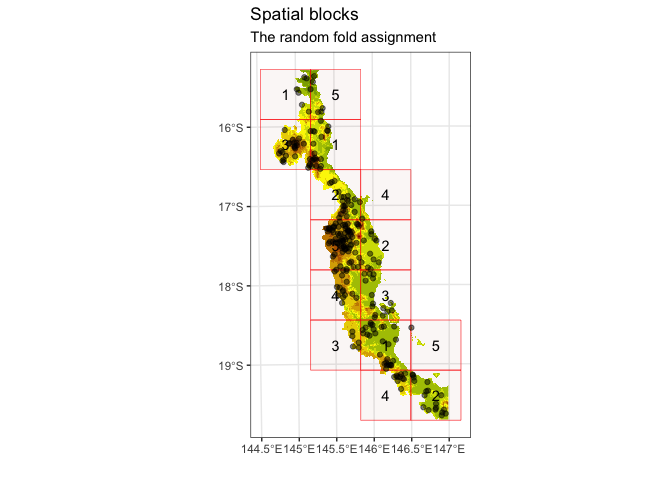### Buffering

The function buffering generates spatially separated training and testing folds by considering buffers of specified distance around each observation point. This approach is a form of leave-one-out cross-validation. Each fold is generated by excluding nearby observations around each testing point within the specified distance (ideally the range of spatial autocorrelation). In this method the test set never directly abuts a training presence or absence.

When working with presence-background (presence and pseudo-absence) data (specified by spDataType argument), only presence records are used for specifying the folds. Consider a target presence point. The buffer is defined around this target point, using the specified range (theRange). The testing fold comprises the target presence point and all background points within the buffer. Any non-target presence points inside the buffer are excluded. All points (presence and background) outside of buffer are used for training set. The method cycles through all the presence data, so the number of folds is equal to the number of presence points in the dataset.

For presence-absence data, folds are created based on all records, both presences and absences. As above, a target observation (presence or absence) forms a test point, all presence and absence points other than the target point within the buffer are ignored, and the training set comprises all presences and absences outside the buffer. Apart from the folds, the number of training-presence, training-absence, testing-presence and testing-absence records is stored and returned in the records table. If species = NULL (no column with 0s and 1s is defined), the procedure is like presence-absence data. All other types of data (continuous, count or multi-class response) should be used like this.

# buffering with presence-absence data
bf1 <- buffering(speciesData = pa_data,
theRange = 70000,
species = "Species", # to count the number of presences and absences/backgrounds
spDataType = "PA", # presence-absence  data type
progress = TRUE)

In the following buffering example, presence-background data are used. As explained above, by default the background data within any target point will remain in the testing fold. This can be changed by setting addBG = FALSE (this option only works when spDataType = "PB"; note the default value is "PA").

# buffering with presence-background data
bf2 <- buffering(speciesData = pb_data, # presence-background data
theRange = 70000,
species = "Species",
spDataType = "PB", # presence-background data type
progress = TRUE)

### Environmental block

The function envBlock uses clustering methods to specify sets of similar environmental conditions based on the input covariates. Species data corresponding to any of these groups or clusters are assigned to a fold.

As k-means algorithms use Euclidean distance to estimate clusters, the input covariates should be quantitative variables. Since variables with wider ranges of values might dominate the clusters and bias the environmental clustering (Hastie et al., 2009), all the input rasters are first standardized within the function. This is done either by normalizing based on subtracting the mean and dividing by the standard deviation of each raster (the default) or optionally by standardizing using linear scaling to constrain all raster values between 0 and 1. By default, the clustering is done in the raster space. In this approach, the clusters will be consistent throughout the region and across species (in the same region). However, this may result in cluster(s) that cover none of the species records especially when species data is not dispersed throughout the region or the number of clusters (k or folds) is high. In this case, the number of folds is less than the specified k. If rasterBlock = FALSE, the clustering will be done based only on the values of the predictors at the species presence and absence/background points. In this case, and the number of the folds will be the same as k.

Note that the input raster layer should cover all the species points, otherwise an error will rise. The records with no raster value should be deleted prior to the analysis.

# environmental clustering
eb <- envBlock(rasterLayer = awt,
speciesData = pa_data,
species = "Species",
k = 5,
standardization = "standard", # rescale variables between 0 and 1
rasterBlock = FALSE,
numLimit = 50)

## The effective range of spatial autocorrelation

To support a first choice of block size, prior to any model fitting, package blockCV includes the option for the user to look at the existing autocorrelation in the predictors, as an indication of landscape spatial structure in their study area. The tool does not suggest any absolute solution to the problem, but serves as a guide to the user. The function works by automatically fitting variograms to each continuous raster and finding the effective range of spatial autocorrelation. Variogram is a fundamental geostatistical tool for measuring spatial autocorrelation. It does so by assessing variability between all pairs of points (O’Sullivan and Unwin, 2010). It provides information about the effective range of spatial autocorrelation which is the range over which observations are independent.

sac <- spatialAutoRange(rasterLayer = awt,
sampleNumber = 5000,
doParallel = TRUE,
showPlots = TRUE)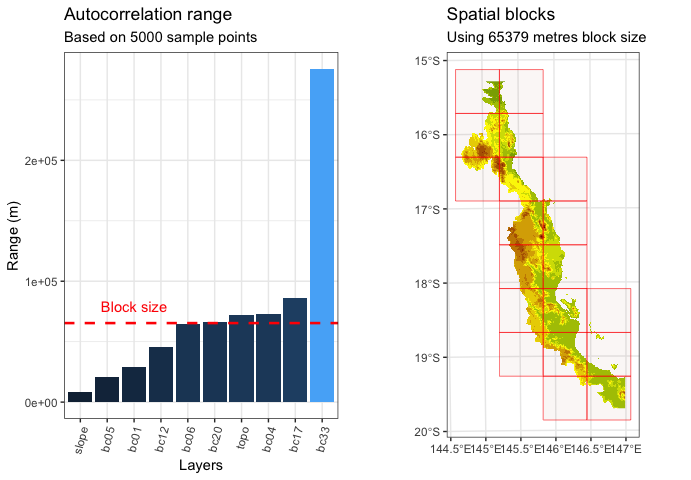The plotted block size is based on the median of the spatial autocorrelation ranges. This could be as the minimum block size for creating spatially separated folds. Variograms are computed taking a number of random points (5000 as default) from each input raster file, using parallel processing to speed up the computation (optional). The variogram fitting procedure is done using automap package (Hiemstra et al., 2009), using the isotropic variogram and assuming the data meets the geostatistical criteria e.g. stationarity.

The output object of this function is an SpatialAutoRange object, an object of class S3.

# class of the output result
class(sac)
##  "SpatialAutoRange"

To see the details of the fitted variograms:

# summary statistics of the output
summary(sac)
## Summary statistics of spatial autocorrelation ranges of all input layers:
##    Min. 1st Qu.  Median    Mean 3rd Qu.    Max.
##    8364   33504   65379   74176   72587  275785
##    layers      range
## 9   slope   8364.193
## 3    bc05  20703.889
## 1    bc01  29393.558
## 5    bc12  45836.241
## 4    bc06  64544.464
## 7    bc20  66213.604
## 10   topo  71668.766
## 2    bc04  72893.585
## 6    bc17  86351.685
## 8    bc33 275785.047

To visualise them (this needs the automap package to be loaded):

library(automap)

plot(sac$variograms[])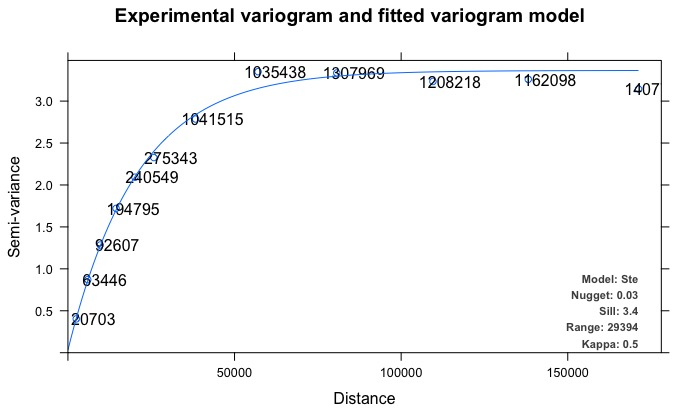## Visualisation tools Package blockCV provides two major visualisation tools for graphical exploration of the generated folds and assisting in block size selection. These tools have been developed as local web applications using R-package shiny. With rangeExplorer, the user can choose among block sizes in a specified range, visualise the resulting blocks interactively, viewing the impact of block size on number and arrangement of blocks in the landscape (and optionally on the distribution of species data in those blocks). The foldExplorer tool displays folds and the number of records in each fold; it works for all three blocking methods. # explore generated folds foldExplorer(blocks = sb, rasterLayer = awt, speciesData = pa_data) # explore the block size rangeExplorer(rasterLayer = awt) # the only mandatory input # add species data to add them on the map rangeExplorer(rasterLayer = awt, speciesData = pa_data, species = "Species", rangeTable = NULL, minRange = 30000, # limit the search domain maxRange = 100000) Note that the interactive plots cannot be shown here, as they require opening an external window or web browser. When using rangeExplorer, slide to the selected block size, and click Apply Changes to change the block size. ## Evaluating SDMs with block cross-validation: examples In this section, we show how to use the folds generated by blockCV in the previous sections for the evaluation of species distribution models constructed on the species data available in the package. The blockCV stores training and testing folds in three different formats. The common format for all three blocking strategies is a list of the id of observations in each fold. For spatialBlock and envBlock (but not buffering), the folds are also stored in a matrix format suitable for the biomod2 package and a vector of fold’s number for each observation. This is equal to the number of observation in species spatial data. These three formats are stored in the blocking objects as folds, biomodTable and foldID respectively. We show three modelling examples which cover both the use of presence-absence and presence-background methods. ### Evaluating presence-background models #### maxnet The code below shows how to evaluate a presence-background model, where maxnet package is used for model fitting. maxnet is a newly developed package by Phillips et. al., (2017) to model species distributions from occurrences and environmental variables, using glmnet for model fitting. The maxnet package is the implementation of Maxent software in R programming language. # loading the libraries library(maxnet) library(precrec) # library(ggplot2) # extract the raster values for the species points as a dataframe mydata <- raster::extract(awt, pb_data) mydata <- as.data.frame(mydata) # create a vector of 1 (for presence) and 0 (for background samples) pb <- pb_data$Species

# extract the folds in spatialBlock object created
# in the previous section (with presence-background data)
# the foldID only works for spatialBlock and envBlock folds
folds <- sb2$foldID # create an empty vector to store the AUC of each fold AUCs <- vector(mode = "numeric") for(k in seq_len(5)){ # extracting the training and testing indices # this way only works with foldID trainSet <- which(folds != k) # training set indices testSet <- which(folds == k) # testing set indices # fitting a maxent model using linear, quadratic and hinge features mx <- maxnet(p = pb[trainSet], data = mydata[trainSet, ], maxnet.formula(p = pb[trainSet], data = mydata[trainSet, ], classes = "default")) testTable <- pb_data[testSet, ] # a table for testing predictions and reference data testTable$pred <- predict(mx, mydata[testSet, ], type = "cloglog") # predict the test set
# calculate area under the ROC curve
precrec_obj <- evalmod(scores = testTable$pred, labels = testTable$Species)
AUCs[k] <- auc(precrec_obj)[1,4] # extract AUC-ROC
}

# print the mean of AUCs
print(mean(AUCs))
##  0.8664762

### Evaluating presence-absence models

#### randomForest

In the second example, we use blocking for evaluating a presence-absence model created using the Random Forest algorithm. Folds generated by buffering function are used here (a training and testing fold for each record).

Note that with buffering using presence-absence data or with species = NULL, there is only one point in each testing fold, and therefore AUC cannot be calculated for each fold separately. Instead, the value of each point is first predicted, and then a unique AUC is calculated for the full set of predictions.

# loading the libraries
library(randomForest)
library(precrec)

# extract the raster values for the species points as a dataframe
mydata <- raster::extract(awt, pa_data, df = TRUE)
# adding species column to the dataframe
mydata$Species <- as.factor(pa_data$Species)
# remove extra column (ID)
mydata <- mydata[,-1]

# extract the foldIDs in SpatialBlock object
# created in the previous section
# the folds (list) works for all three blocking strategies
folds <- bf1$folds # create a data.frame to store the prediction of each fold (record) testTable <- pa_data testTable$pred <- NA

for(k in seq_len(length(folds))){
# extracting the training and testing indices
# this way works with folds list (but not foldID)
trainSet <- unlist(folds[[k]]) # training set indices
testSet <- unlist(folds[[k]]) # testing set indices
rf <- randomForest(Species~., mydata[trainSet, ], ntree = 250) # model fitting on training set
testTable$pred[testSet] <- predict(rf, mydata[testSet, ], type = "prob")[,2] # predict the test set } # calculate Area Under the ROC and PR curves and plot the result precrec_obj <- evalmod(scores = testTable$pred, labels = testTable$Species) autoplot(precrec_obj)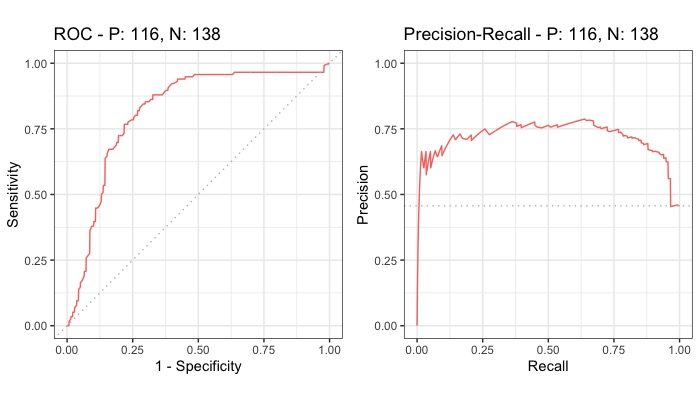#### biomod2 Package biomod2 (Thuiller et al., 2017) is a commonly used platform for modelling species distributions in an ensemble framework. In this example, we show how to use blockCV folds in biomod2. In this example, the DataSplitTable generated by spatialBlock is used to evaluate three modelling methods implemented in biomod2. The DataSplitTable can be generated by both spatialBlock and envBlock functions and it is stored as biomodTable in their output objects. # loading the library library(biomod2) # species occurrences DataSpecies <- read.csv(system.file("extdata", "PA.csv", package = "blockCV")) # the name of studied species myRespName <- "Species" # the presence/absences data for our species myResp <- as.numeric(DataSpecies[,myRespName]) # the XY coordinates of species data myRespXY <- DataSpecies[,c("x","y")] # change the RasterBrick to RasterStack awt <- stack(awt) # 1. Formatting Data myBiomodData <- BIOMOD_FormatingData(resp.var = myResp, expl.var = awt, # explanatory raster data resp.xy = myRespXY, resp.name = myRespName, na.rm = TRUE) # 2. Defining the folds for DataSplitTable # note that biomodTable should be used here not folds DataSplitTable <- sb$biomodTable # use generated folds from spatialBlock in previous section

# 3. Defining Models Options using default options.
myBiomodOption <- BIOMOD_ModelingOptions()

# 4. Model fitting
myBiomodModelOut <- BIOMOD_Modeling( myBiomodData,
models = c('GLM','MARS','GBM'),
models.options = myBiomodOption,
DataSplitTable = DataSplitTable, # blocking folds
VarImport = 0,
models.eval.meth = c('ROC'),
do.full.models=FALSE,
modeling.id="test")
# 5. Model evaluation
# get all models evaluation
myBiomodModelEval <- get_evaluations(myBiomodModelOut)
myBiomodModelEval["ROC","Testing.data",,,]

Note that the result of this section (biomod model evaluation) is not shown.